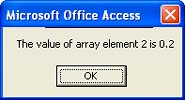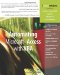# Working with Array Elements

 < Day Day Up >

After declaring an array variable, there are two ways to manipulate that array's elements:

• You can define the array elements

• You can refer to the array elements

Defining and referencing array elements is similar to doing so with any other variables. Just remember, you have to work within the context of the array's index values.

#### Defining Array Elements

Declaring an array is only half the job. The next step is to actually store the elements in it. To do so, use the simple syntax

` `

` arrayvariable(index) = value `

where index identifies the element's position within the array and value is any valid value or expression that fits the declared data type. Following the previous example

` `

` Dim arrInteger(1 To 4) As Integer `

index can be only the values 1, 2, 3, and 4, but value can be any valid integer. For instance, all the following statements are valid:

• arrInteger(1) = 100

• arrInteger(2) = -200

• arrInteger(3) = 3

• arrInteger(4) = 40

The previous statements set arrInteger's four elements to the values 100, 200, 3, and 40, respectively.

Similarly, the following procedure sets the value of four array elements to . 1, . 2, . 3, and . 4:

` `

` Public Sub ArrayExample1()   Dim intCounter As Integer   Dim arrExample(1 To 4) As Variant   For intCounter = 1 To 4     arrExample(intCounter) = intCounter * 0.1   Next End Sub `

The lower bound is 1 and the upper bound is 4. The For…Next statement loops once for each intCounter value (1 through 4). During each loop, intCounter equals an index value. (This won't always be the case.) The procedure multiples the index value by .10 and assigns the resulting value to the corresponding element. For instance, the first time through, intCounter equals 1, so the definition statement evaluates as follows

` `

` arrExample (1) = 1 * 0.1 arrExample (1) = .1 `

The second time through the loop, intCounter equals 2, so arrExample(2) equals .2 (2 * 0.1), and so on.To learn how to use the For…Next statement, see "Using For…Next," p. 95.

#### Referencing Array Elements

The previous Sub function assigned a value to each of the array's four elements. What you need to know now is how to access those values. You access array elements similarly to the way you defined them you use the index values in the form

` `

` variable = arrayvariable(index) `

For instance, to refer to any of the elements in arrExample from the previous example, refer to the appropriate element index value as follows:

` `

` arrExample(1) arrExample(2) arrExample(3) arrExample(4) `

To illustrate referencing an element, use the following Sub procedure to pass a value to the procedure and then display the corresponding array element in a message box:

` `

` Public Sub ArrayExample2(ele As Integer)   Dim intCounter As Integer   Dim arrExample(1 To 4) As Variant   For intCounter = 1 To 4     arrExample(intCounter) = intCounter * 0.1   Next   MsgBox "The value of array element " & _    ele & " is " & arrExample(ele), vbOKOnly End Sub `

To see the procedure in action, launch the VBE, open a blank module, and enter the procedure. Or, open Chapter7 in the TimeTrack.mdb sample database. Then, enter the following statement in the Immediate window

` `

` arrayExample2(x) `

where x is the value 1, 2, 3, or 4, and then press Enter. (There's no error-handling in this procedure, so if you enter a value other than 1 through 4, the message box will display an incomplete message.) The procedure sets all four array elements, but returns only the value of the element represented by the passed value. Figure 7.1 shows the result of passing the value 2 to the procedure. Click OK to clear the message box and return to the VBE.

##### Figure 7.1. Use the index value to reference an array's elements.To learn how to include error handling in your VBA procedures, see "Implementing Error Handling," p. 52.

 < Day Day Up >Automating Microsoft Access with VBA
ISBN: 0789732440
EAN: 2147483647
Year: 2003
Pages: 186